# Supplementary Methods Statistical analysis by normal mixture model-based clustering

 Supplementary Methods Statistical analysis by normal mixture model-based clustering Firstly, the normal mixture model-based clustering method using the SMC1 proportion and KAP1 proportion was applied separately. Then the normal mixture model-based clustering method considering the SMC1 proportion and KAP1 proportion was applied simultaneously. For SMC1 only, the estimated normal mixture distribution is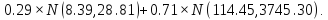Two clusters are defined using the optimal cut-off of 20.9. For KAP1 only, the estimated normal mixture distribution is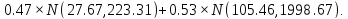Two clusters are defined using the optimal cut-off of 54.64. For SMC1 and KAP1 together, the estimated normal mixture distribution is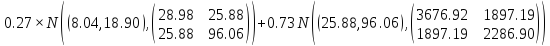. The boundary of the two clusters is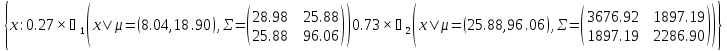, where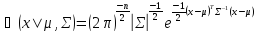. Somatic mutation detection from captured DNA sequencing The program evaluates each aligned base and its base quality value at each position to indicate putative single-nucleotide variations (SNVs) and short insertions/deletions (INDELs), and their corresponding SNV probability value (PSNV). Base quality values were converted to base probabilities corresponding to every one of the four possible nucleotides. Using a Bayesian formulation, a PSNV (or INDEL probability value, as appropriate) was calculated as the likelihood that multiple different alleles are present between the reference genome sequence and the reads aligned at that position. If the probability value exceeded a pre-specified threshold, the SNV or INDEL candidate was reported in the output. In this study, a certain PSNV cutoff value (say 0.9) was used to define a high-confidence SNV or short INDEL candidate. All known SNVs/INDELs were filtered out in UCSC dbSNP 142 (human) and 1000 human genome project SNP database. The somatic status of each SNVs (or INDELS) was determined by comparing the genotypes and its likelihood between matched normal and tumor samples. Directory: bcjShare with your friends: